# Overview

The Decision Tree Classification Forecast Processor generates a forecast for a categorical dependent variable based on a learned decision tree.

In the decision tree algorithm, the original input data is split into various partitions based on an impurity criterion. The impurity measure splits the data by creating homogeneous data points within one node with regard to the output variable.

The dependent variable is predicted using the trained dataset represented by the classification tree. More specifically, this prediction is extracted from the leaves carrying the information of both the independent variable's range (interval, set of values) and the dependent variable's assigned label.

Further Information about decision trees can be found in the following link.

# Input

The processor requires two input datasets. The first input port (the one on the left) corresponding to the training dataset (this data should be already labeled). The second input port (the one on the right) corresponding to the test dataset.

It goes without saying that the training and the test datasets should have the same schema.

# Configuration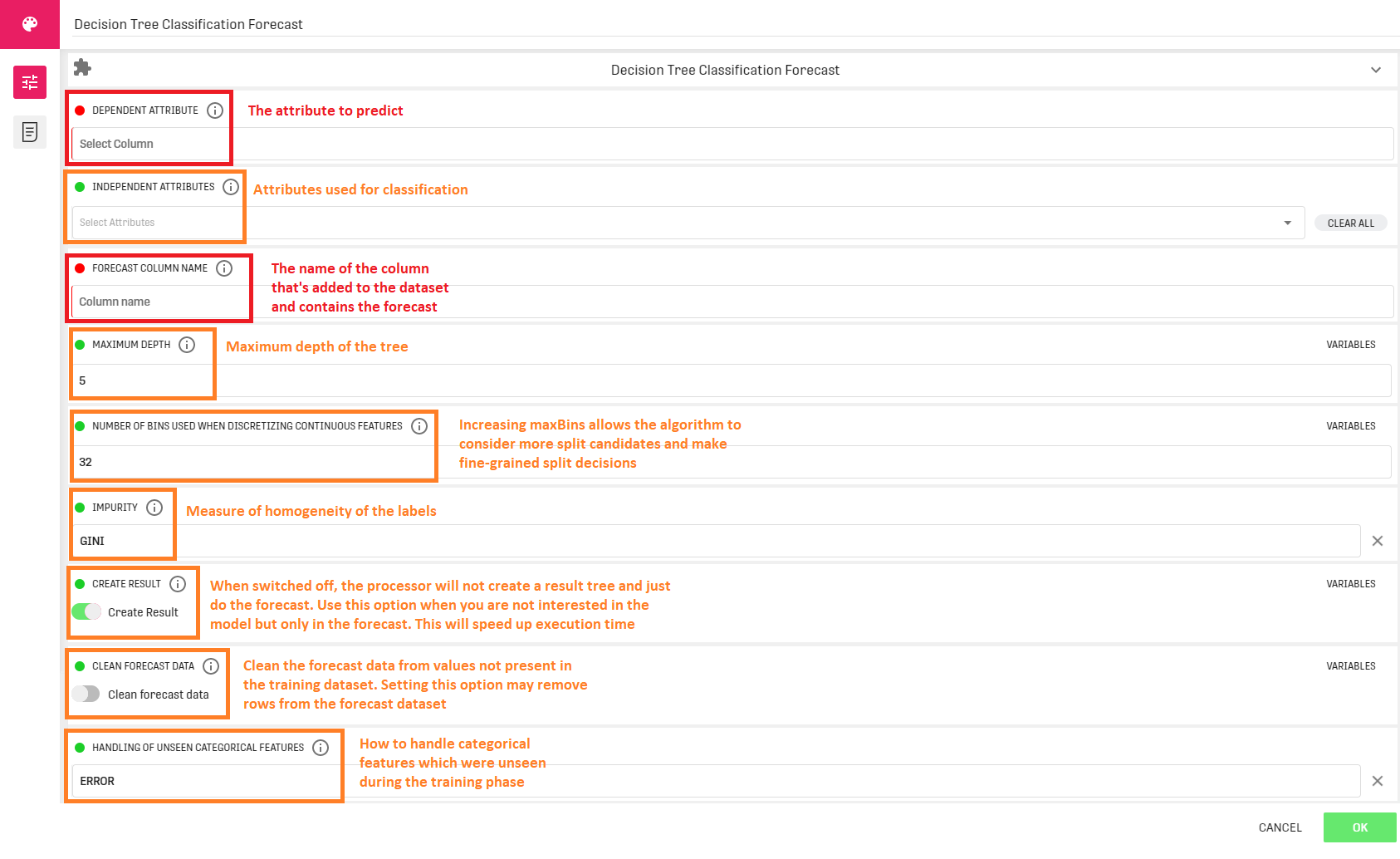The last parameter (Handling of unseen categorical features) has three options:

• KEEP: creates one new category for all unseen values
• ERROR: fails if unseen values occur
• SKIP: ignores the unseen values

# Output

The Decision Tree Classification Processor can provide two different outputs:

• A decision tree: Based on the training dataset. This tree can be accessed through the decision tree classification processor under the tab "Results".
• A forecast table: The test dataset with the added "prediction" column. It can be viewed via the result table linked to the processor's output.

# Example

In this example, the input dataset represents information about train passengers (Name, Class, Age...). The goal is to build a decision tree that predicts the passenger's sex using the fare column.

## Example Input

We used a Horizontal Split Processor to split the input dataset (418 entries) into two different datasets: The training dataset containing 80% of the input data (334 entries) and the Test dataset containing the remaining 20% (84 entries).

Training Dataset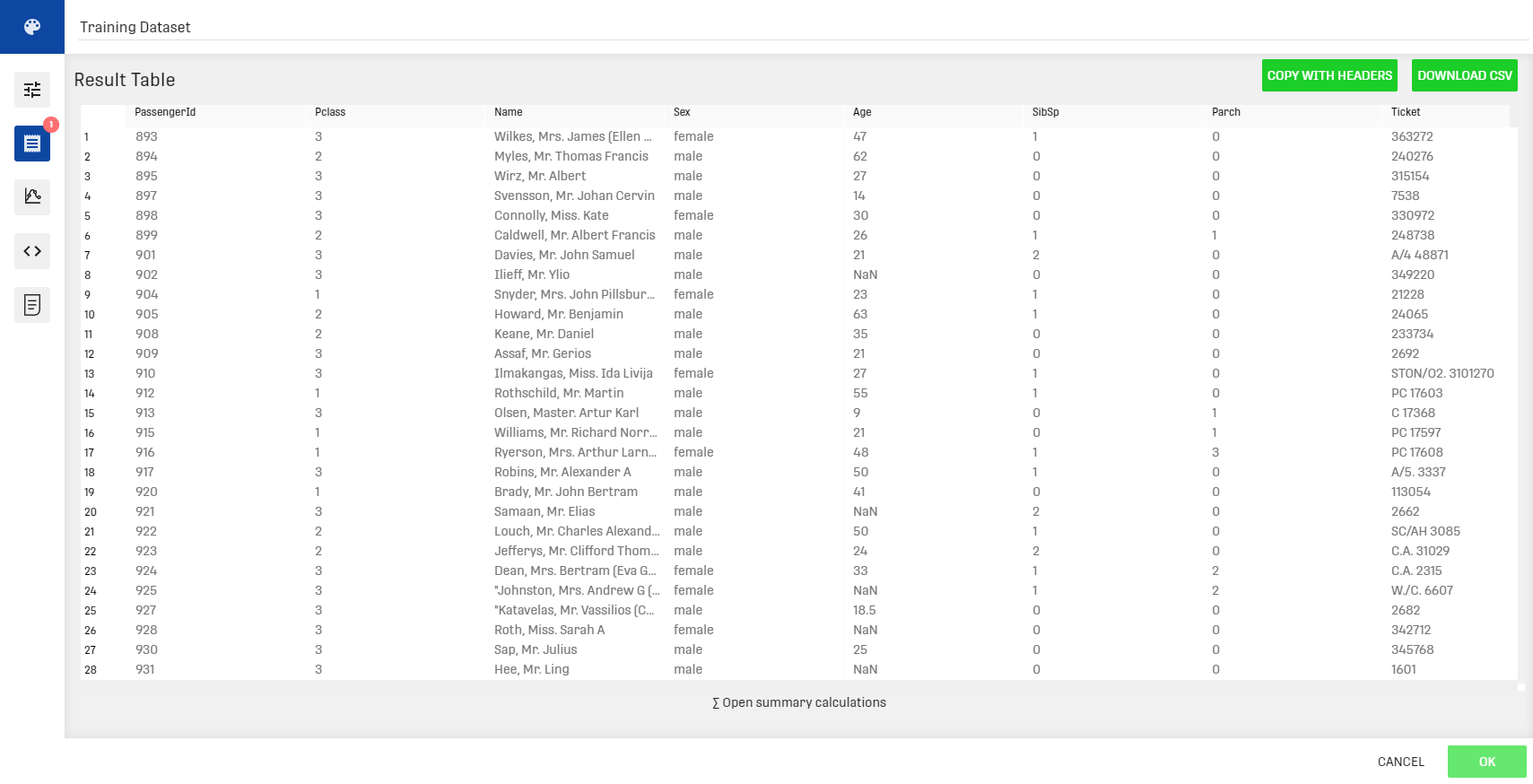## Example Configuration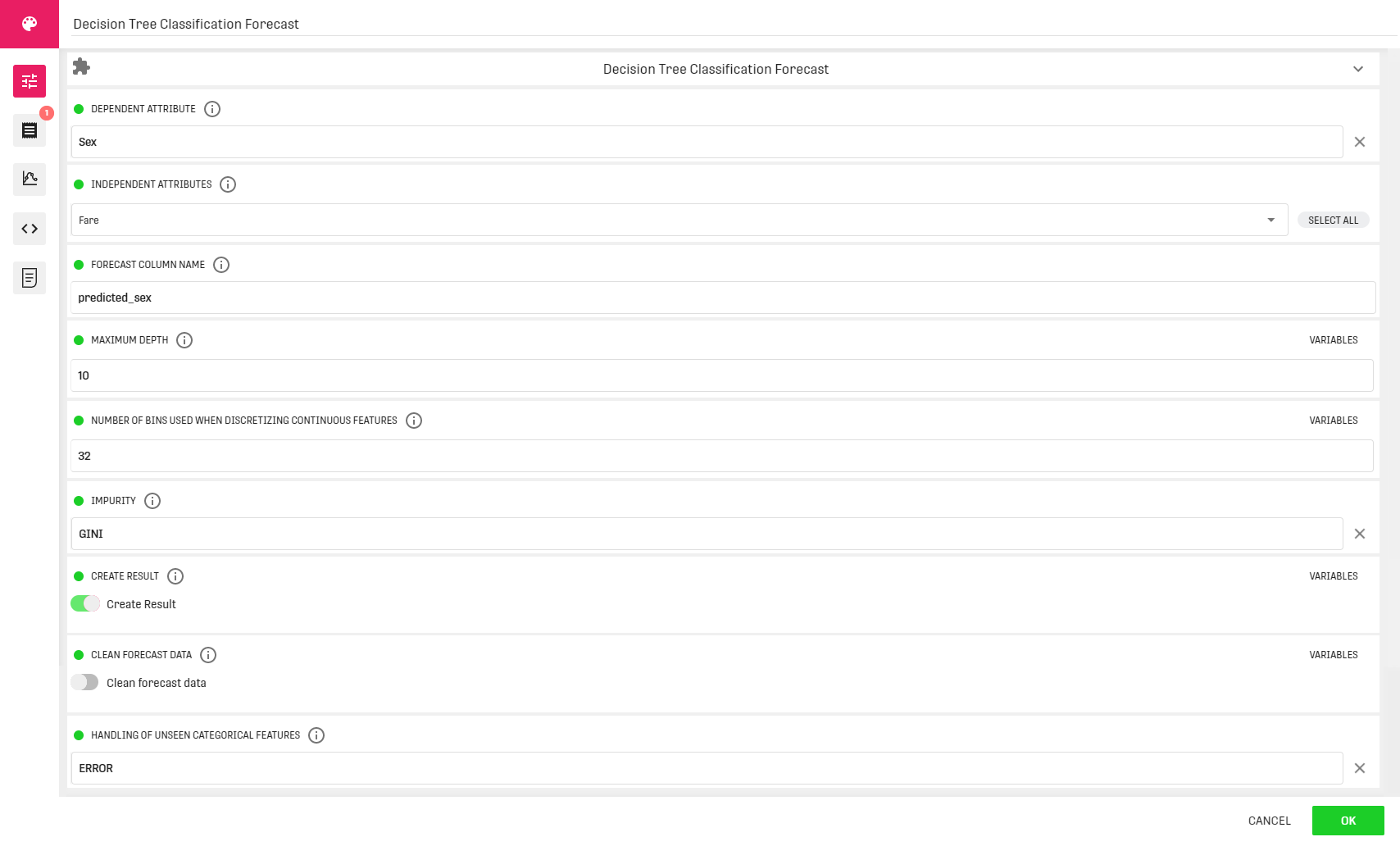Note that we are using the GINI Impurity in this example. Using the Entropy impurity will have the same configuration.

## Result

decision tree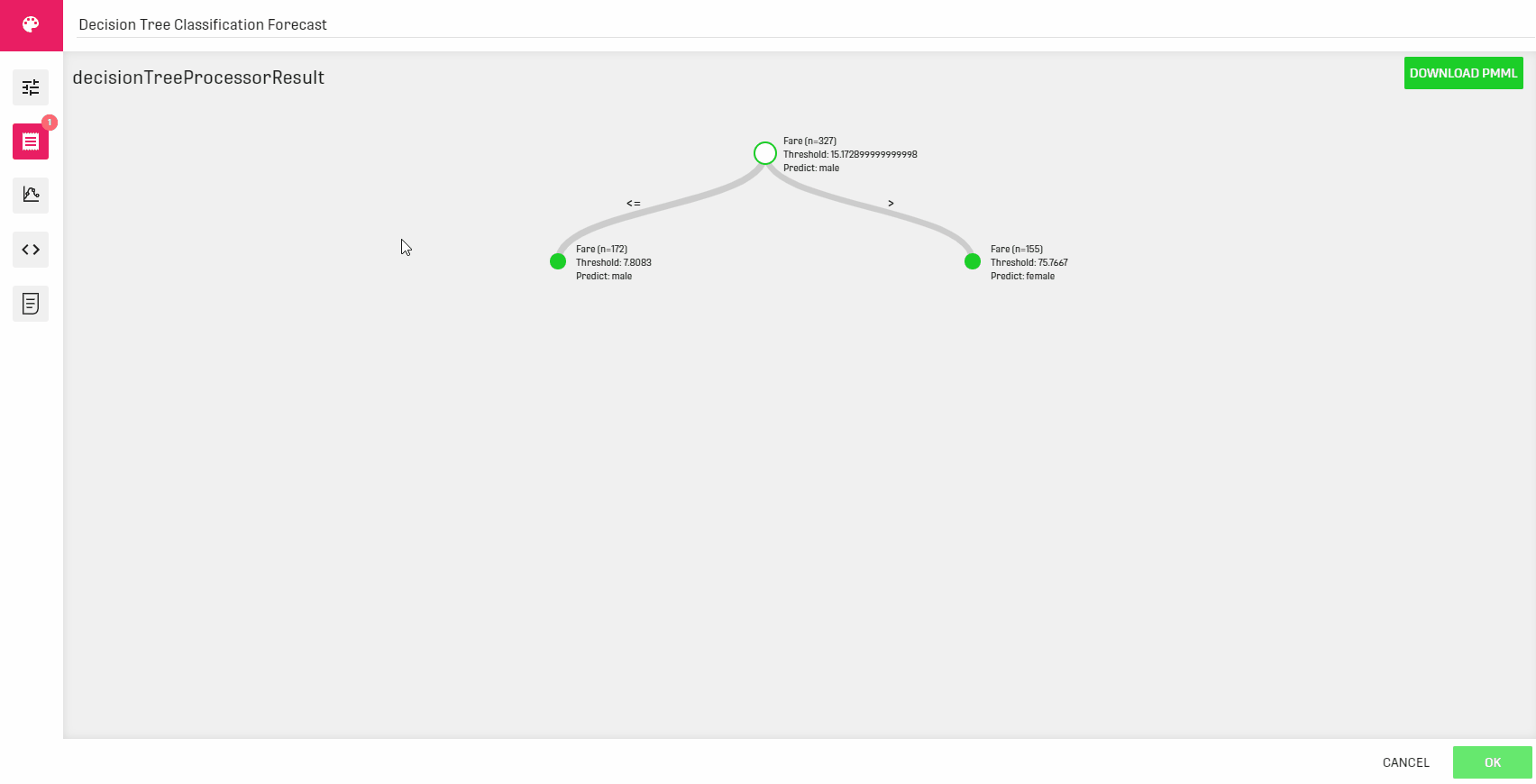Result table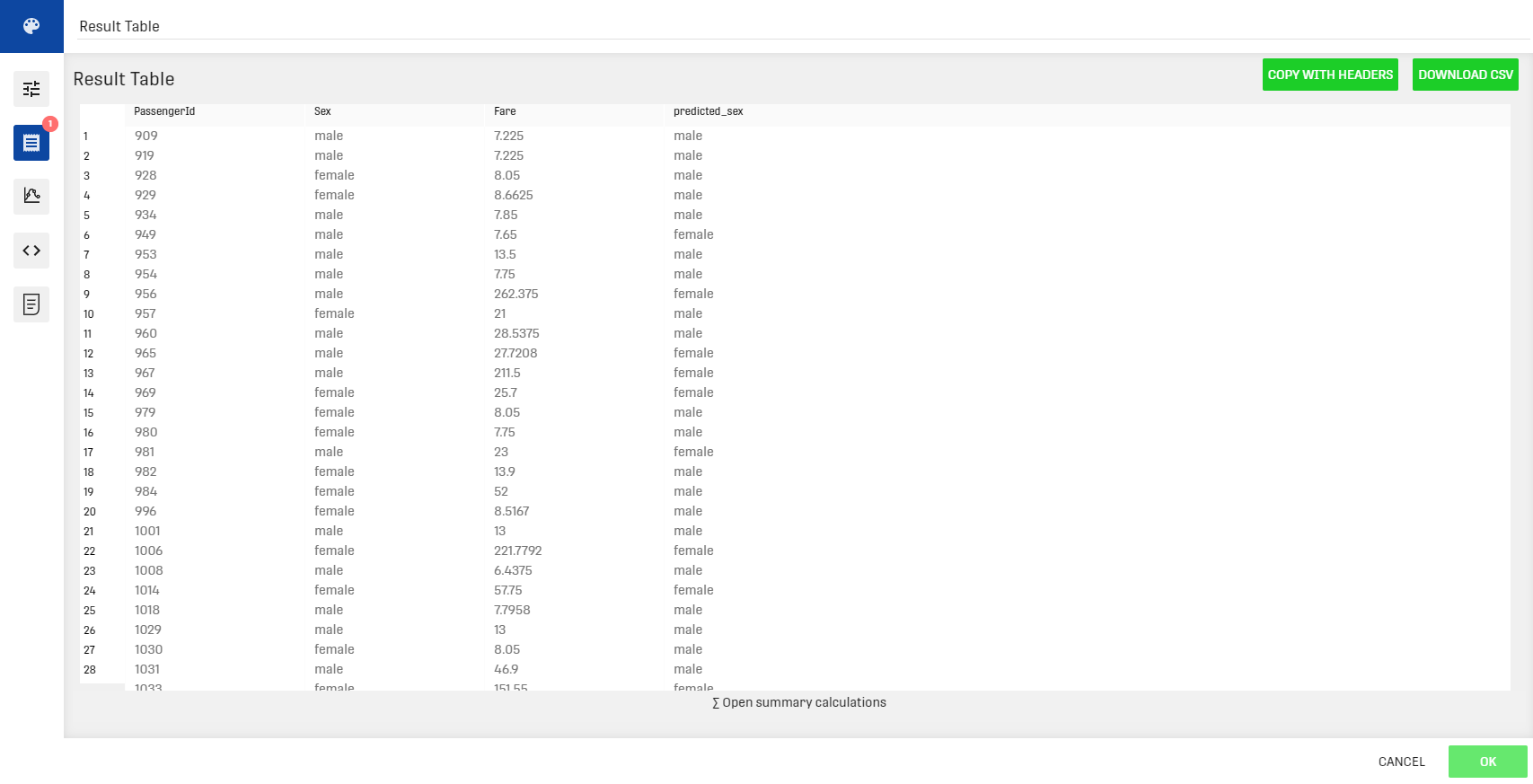Note that the predicted labels don't exactly match the actual labels. With that being said a training data with 400 entries isn't sufficient. The training dataset needs to be of a considerable size in order to have a more accurate result and reduce the error ratio.

# Related Articles

Decision Tree Classification Processor

Decision Tree Regression Processor

Decision Tree Regression Forecast Processor